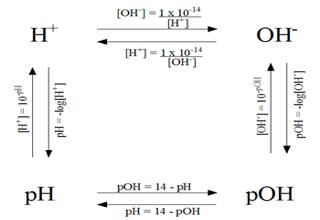Chemistry

# pOH Measurement

A measurement of the hydroxide ion concentration, similar to pH, is called pOH.

The pOH of a solution is defined as the negative logarithm of the molar hydroxide- ion concentration as follows:

pOH = -log10 [OH-]

Because KW = [H+] x [OH] = 1.0 x 10-14 at 25°C, one can demonstrate that

pH + pOH = 14.00Example

A solution has a hydroxide-ion concentration of 1.9 x 10-3 M. What is the pH of the solution?

Firstly, calculate the pOH:

pOH = -log10 (1.9 x 10-3) = 2.72

Then, calculate the pH:

pH = 14.00 — 2.72 = 11.28